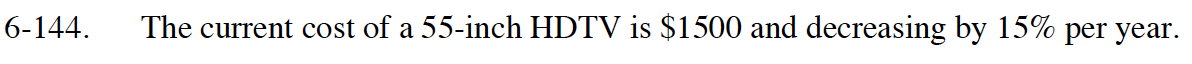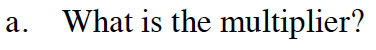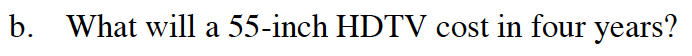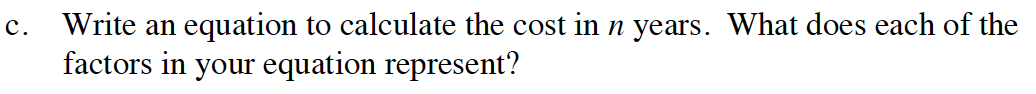Home > GB8I > Chapter cc46 > Lesson cc46.4.2 > Problem6-144

6-144.
1. The current cost of a 55-inch HDTV is $1500 and decreasing by 15% per year. Homework Help ✎ 1. What is the multiplier? 2. What will a 55-inch HDTV cost in four years? 3. Write an equation to calculate the cost in n years. What does each of the factors in your equation represent?If the cost decreased by 15% each year, the new cost would be 85% of the previous year. 0.85In one year the cost will be$1500(0.85).
In two years the cost will be [\$1500(0.85)](0.85).
Continue this pattern.Use the pattern in part (b) to write your equation.